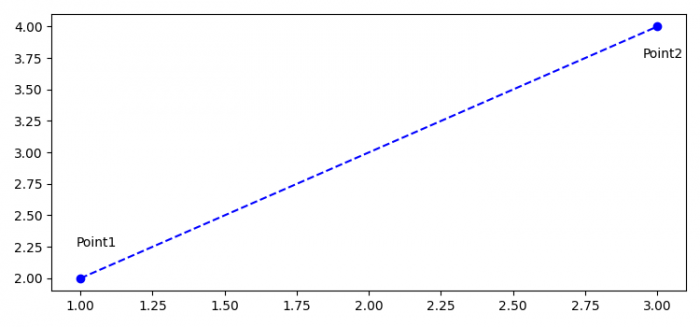# How do you create line segments between two points in Matplotlib?

MatplotlibPythonData Visualization

#### Python Data Science basics with Numpy, Pandas and Matplotlib

Most Popular

63 Lectures 6 hours

#### Data Visualization using MatPlotLib & Seaborn

11 Lectures 4 hours

#### MatPlotLib with Python

9 Lectures 2.5 hours

To create line segments between two points in matplotlib, we can take the following steps

• Set the figure size and adjust the padding between and around the subplots.
• To make two points, create two lists.
• Extract x and y values from point1 and point2.
• Plot x and y values using plot() method.
• Place text for both the points.
• To display the figure, use show() method.

## Example

import matplotlib.pyplot as plt
plt.rcParams["figure.figsize"] = [7.50, 3.50]
plt.rcParams["figure.autolayout"] = True
point1 = [1, 2]
point2 = [3, 4]
x_values = [point1, point2]
y_values = [point1, point2]
plt.plot(x_values, y_values, 'bo', linestyle="--")
plt.text(point1-0.015, point1+0.25, "Point1")
plt.text(point2-0.050, point2-0.25, "Point2")
plt.show()

## Output